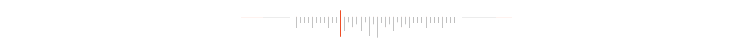# 古人是如何寻找到π的？

π的历史简介

π为什么是常数？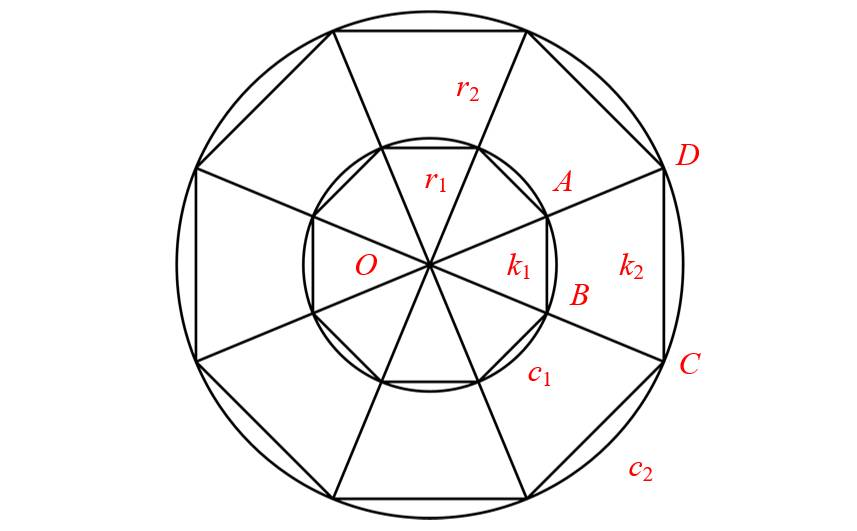Archimedes

287-212 BC

(图片来源: Wikipedia)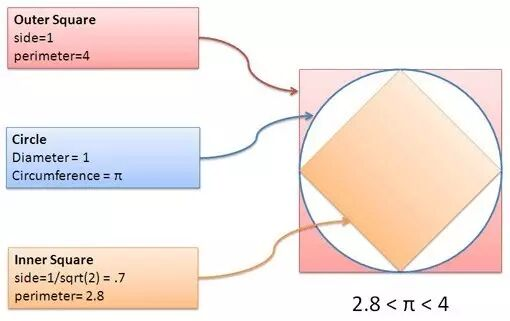(图片来源: betterexplained)

P4=1×4=4

p4≈0.7×4=2.8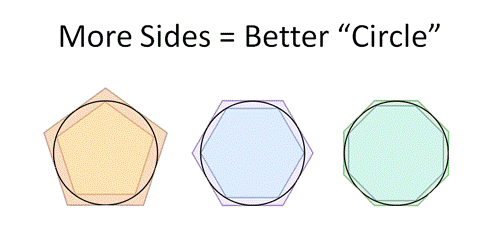Archimedes pi

(图片来源: Wikipedia)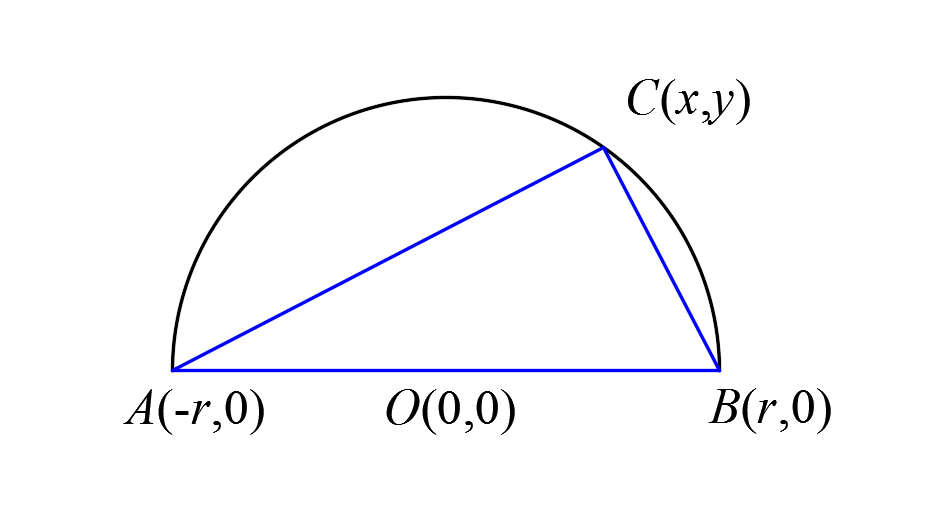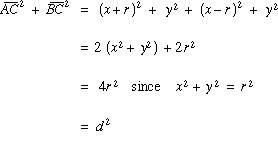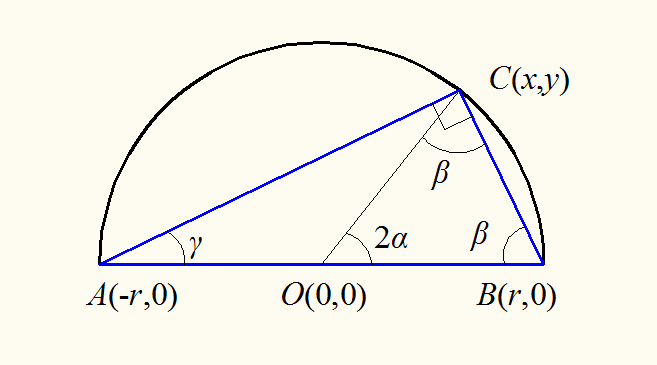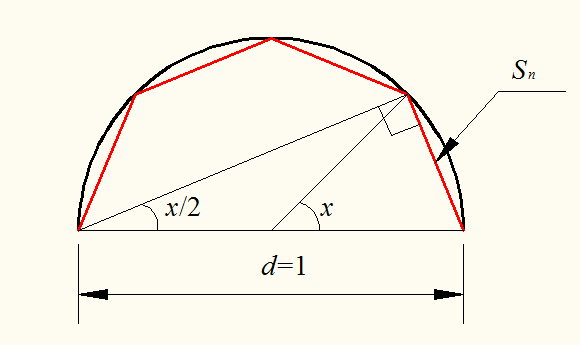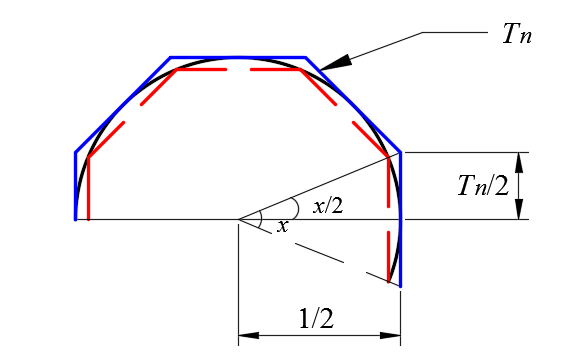p4=4×sin[(360°/4)/2]= 2.8284271247

P4=4×tan[(360°/4)/2]=4

p8=8×sin[(360°/8)/2]= 3.0614674589

P8=8×tan[(360°/8)/2]= 3.313708499

pn=n×sin[(360°/n)/2]

Pn =n×tan[(360°/n)/2]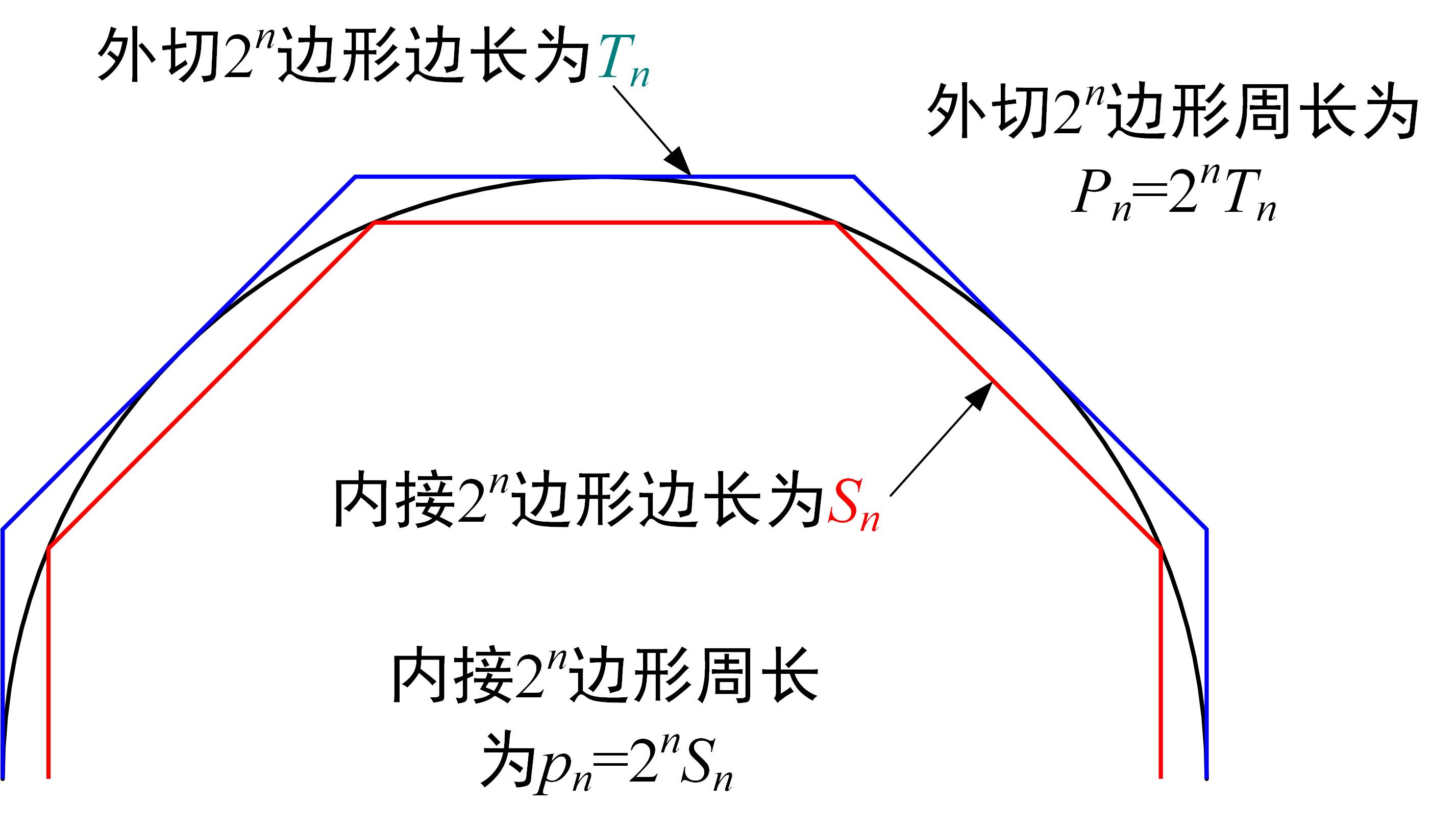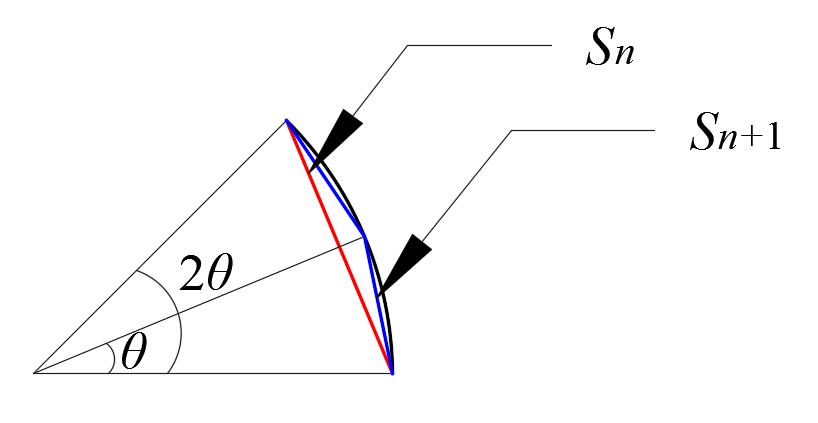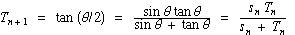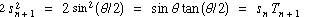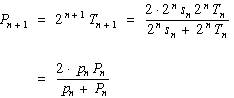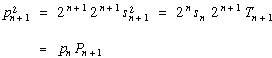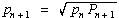Pn+1pnPn的“调和平均数”；

pn+1pnPn+1的“几何平均数”。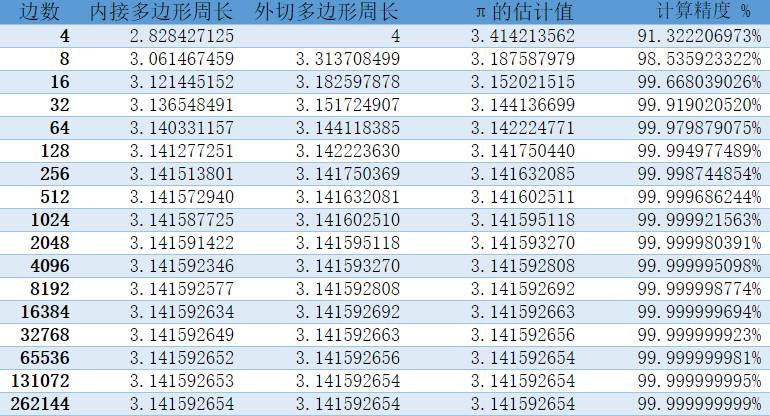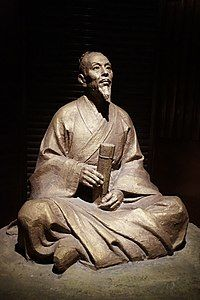(图片来源: Wikipedia)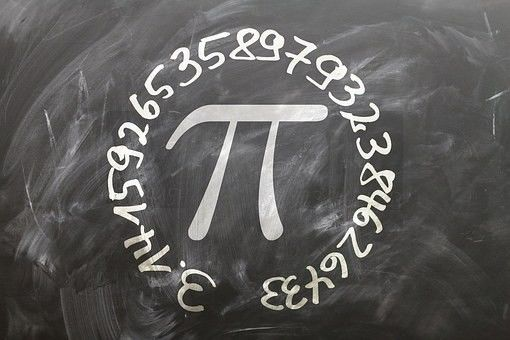Reference

Archimedes

https://en.wikipedia.org/wiki/Archimedes

Zu Chongzhi

https://en.wikipedia.org/wiki/Zu_Chongzhi

Prehistoric Calculus: Discovering Pi

https://betterexplained.com/articles/prehistoric-calculus-discovering-pi/